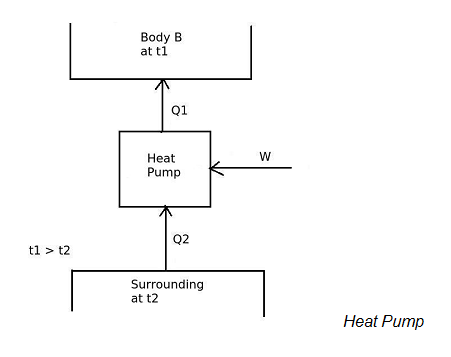# COP of a heat pump is.

## COP of a heat pump is

a. always less than infinity (COP < ∞ )
b. always less that 1 (COP < 1)
c. always equals to 1 (COP = 1)
d. always equals to infinity (COP = ∞ )

Correct Answer : a. always less than infinity (COP < ∞ )

Explanation :Heat pump is a device, which operates in a cycle and maintains the temperature a particular body higher than the surrounding temperature. The desired effect is to maintain the temperature of a body higher than the surrounding temperature. Therefore COP of the heat pump is given by the equation,
[COP]H.P. = Desired effect / Work input
[COP]H.P = Q1 / W

According to the Clausius statement of second law of thermodynamics, it is impossible to construct a heat pump, operating in a cycle, whose sole effect is to transfer of heat from a cooler body to a hotter body, without expending any work input.
Therefore, work input W will never be zero. Thus COP of heat pump is always less than infinity (COP < ∞)